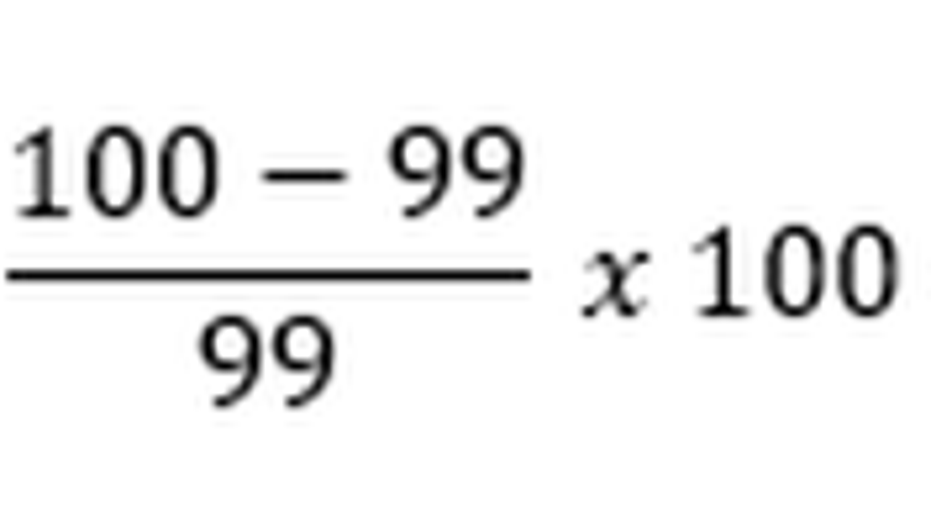# How to Calculate the Percentage Return of a Treasury BillA Treasury bill, or T-bill, is a short-term government debt security with a maturity of less than one year. Unlike many other debt securities that make regular interest payments to investors, Treasury bills yield no interest. Rather, the bills are sold at a discount to their redemption price. For example, a Treasury bill with a face value of \$1,000 might sell for \$985.

Treasury bill prices are expressed on a scale where 100 represents full par value. So, the Treasury bill I just mentioned would have a price of 98.5.

## Calculating the return of a Treasury bill

In order to calculate the return of a Treasury bill, we need to compare its par value to its face value. Additionally, since investment returns are most useful when expressed on an annualized basis, we'll also need to use the maturity period to convert the return to an annual percentage.

So, the first thing you'll need to do is gather some information -- specifically, you'll need to know the Treasury bill's purchase price, date of purchase, and maturity date. From this information, you'll need to determine the number of days until maturity in order to be able to annualize the return.

The first calculation involves subtracting the T-bill's price from 100 and dividing this amount by the price. This figure tells you the T-bill's yield during the maturity period. Multiply this number by 100 to convert to a percentage.

Next, multiply the yield you just calculated by 365 and then divide by the number of days in the maturity period that you determined earlier. This will give you the T-bill's annualized investment return.

To illustrate this, let's take a look at an example. Let's say that you buy a 13-week Treasury bill (91 days to maturity) at a price of 99.0. During the maturity period, we can calculate the T-bill's yield as:

Finally, to annualize the return, we can use our formula to calculate:

This is the number you would use to compare your T-bill to other investment options. By annualizing the return, a 13-week T-bill's return can be easily compared to longer-dated T-bills as well as Treasury bonds, corporate bonds, and other types of fixed-income investments.

This article is part of The Motley Fool's Knowledge Center, which was created based on the collected wisdom of a fantastic community of investors. We'd love to hear your questions, thoughts, and opinions on the Knowledge Center in general or this page in particular. Your input will help us help the world invest, better! Email us atknowledgecenter@fool.com. Thanks -- and Fool on!

The article How to Calculate the Percentage Return of a Treasury Bill originally appeared on Fool.com.

Try any of our Foolish newsletter services free for 30 days. We Fools may not all hold the same opinions, but we all believe that considering a diverse range of insights makes us better investors. The Motley Fool has a disclosure policy.